[Last time](https://forum.azimuthproject.org/discussion/2335/lecture-73-string-diagrams-and-strictification/p1) we talked about string diagrams, which are a great way to draw morphisms in symmetric monoidal categories. But they're the most fun when we have the ability to take a diagram and bend its strings around, converting inputs to outputs or vice versa. And that's what 'compact closed' categories are for!

A **compact closed category** is a symmetric monoidal category \$$\mathcal{C}\$$ where every object \$$x\$$ has a **dual** \$$x^\ast\$$ equipped with two morphisms called the **cap** or **unit**

$\cap_x \colon I \to x \otimes x^\ast$

and the **cup** or **counit**

$\cup_x \colon x^\ast \otimes x \to I$

obeying two equations called the **snake equations**.

You've seen these equations a couple times before! In [Lecture 68](https://forum.azimuthproject.org/discussion/2304/lecture-68-chapter-4-feedback-in-collaborative-design/p1) I was telling you about feedback in co-design diagrams: caps and cups let you describe feedback. I was secretly telling you that the category of feasibility relations was a compact closed category. In [Lecture 71](https://forum.azimuthproject.org/discussion/2315/lecture-71-caps-and-cups-for-enriched-profunctors/p1) I came back to this theme at a higher level of generality. Feasibility relations are just \$$\mathcal{V}\$$-enriched profunctors for \$$\mathcal{V} = \mathbf{Bool}\$$, and in Lecture 71 I was secretly telling you that the category of \$$\mathcal{V}\$$-profunctors is always a compact closed category! But now I'm finally telling you what a compact closed category is in general.

The snake equations are easiest to remember using string diagrams. In a compact closed category we draw arrows on string in these diagrams as well as labeling them by objects. For any object \$$x\$$ a left-pointing wire labelled \$$x\$$ means the same as a right-pointing wire labelled \$$x^\ast\$$. Thus, we draw the cap as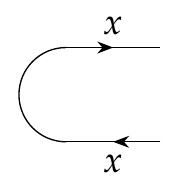This picture has no wires coming in at left, which says that the cap is a morphism from \$$I\$$, the unit object of our symmetric monoidal category. It has two wires doing out at right: the top wire with a right-pointing arrow, stands for \$$x\$$, while the bottom wire with a right-pointing arrow stands for \$$x^\ast\$$, and together these tell us that cap is a morphism to \$$x \otimes x^\ast\$$.

Similarly, we draw the cup as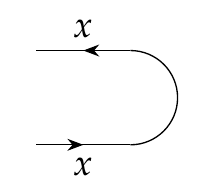and this diagram, to the trained eye, says that the cup is a morphism from \$$x^\ast \otimes x \$$ to \$$I \$$.

In this language, the snake equations simply say that we can straighten out a 'zig-zag':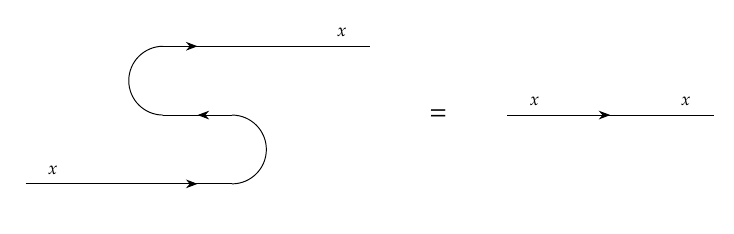or a 'zag-zig':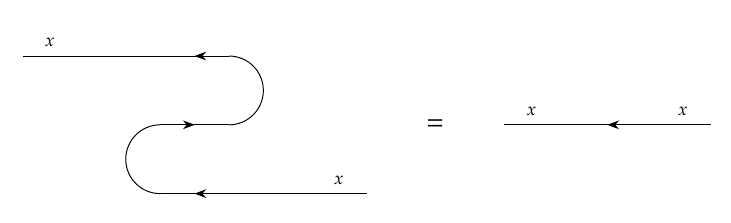If we don't use string diagrams, these equation look more complicated. The first says that this composite morphism is the identity: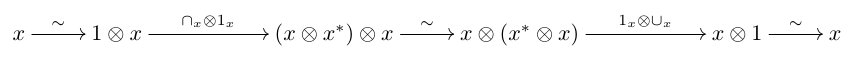where the unnamed isomorphisms are the inverse of the left unitor, the associator and the right unitor. The second says that this composite is the identity: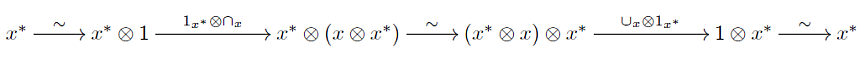where the unnamed isomorphisms are the inverse of the right unitor, the inverse of the associator, and the left unitor. These are a lot less intuitive, I think! One advantage of string diagrams is that they hide associators and unitors, yet let us recover them if we really need them.

If you've faithfully done all the puzzles so far, you've proved the following grand result, which summarizes a lot of this chapter:

**Theorem.** Suppose \$$\mathcal{V}\$$ is a commutative quantale. Then the category \$$\mathbf{Prof}_{\mathcal{V}}\$$ with

* \$$\mathcal{V}\$$-enriched categories as objects

and

* \$$\mathcal{V}\$$-enriched profunctors as morphisms

is compact closed, where tensor product, associator and unitors are defined as in [Lecture 70](https://forum.azimuthproject.org/discussion/2310/lecture-70-tensoring-enriched-profunctors/p1), and the dual, caps and cups are defined as in [Lecture 71](https://forum.azimuthproject.org/discussion/2315/lecture-71-caps-and-cups-for-enriched-profunctors).

But the most famous example of a compact closed category comes from linear algebra! It's the category \$$\mathbf{FinVect}_k\$$, with

* finite-dimensional vector spaces over the field \$$k\$$ as objects

and

* linear maps as morphisms.

If you don't know about [fields](https://en.wikipedia.org/wiki/Field_(mathematics)), you may still know about real vector spaces: that's the case \$$k = \mathbb{R}\$$. There's a [tensor product](https://en.wikipedia.org/wiki/Tensor_product#Tensor_product_of_vector_spaces) \$$V \otimes W\$$ of vector spaces \$$V\$$ and \$$W\$$, which has dimension equal to the dimension of \$$V\$$ times the dimension of \$$W\$$. And there's a [dual](https://en.wikipedia.org/wiki/Dual_space) \$$V^\ast\$$ of a vector space \$$V\$$, which is just the space of all linear maps from \$$V\$$ to \$$k\$$.

Tensor products and dual vector spaces are very important in linear algebra. My main point here is that the profunctors work a lot like linear maps: both are morphisms in some compact closed category! Indeed, the introduction of profunctors into category theory was very much like the introduction of linear algebra in ordinary set-based mathematics. I've tried to hint at this several times: the ultimate reason is that composing profunctors is a lot like multiplying matrices! This is easiest to see for \$$\mathcal{V}\$$-enriched profunctors we've been dealing with. Composing these:

$(\Psi\Phi)(x,z) = \bigvee_{y \in \mathrm{Ob}(\mathcal{Y})} \Phi(x,y) \otimes \Psi(y,z)$

looks just like matrix multiplication, with \$$\bigvee\$$ replacing addition in the field \$$k\$$ and \$$\otimes\$$ replacing multiplication. So it's not surprising that this analogy extends, with the opposite of a \$$\mathcal{V}\$$-enriched category acting like a dual vector space.

If you're comfortable with tensor products and duals of vector spaces, you may want to solidify your understanding of compact closed categories by doing this puzzle:

**Puzzle 283.** Guess what the cap and cup

$$\cap_V \colon k \to V \otimes V^\ast, \qquad \cup_V \colon V^\ast \otimes V \to k$$

are for a finite-dimensional vector space \$$V\$$, and check your guess by proving the snake equations.

Here are some good things to know about compact closed categories:

**Puzzle 284.** Using the cap and cup, any morphism \$$f \colon x \to y \$$ in a compact closed category gives rise to a morphism from \$$y^\ast\$$ to \$$x^\ast\$$. This amounts to 'turning \$$f\$$ around' in a certain sense, and we call this morphism \$$f^\ast \colon y^\ast \to x^\ast \$$. Write down a formula for \$$f^\ast\$$ and also draw it as a string diagram.

**Puzzle 285.** Show that \$$(fg)^\ast = g^\ast f^\ast \$$ for any composable morphisms \$$f\$$ and \$$g\$$, and show that \$$(1_x)^\ast = 1_x \$$ for any object \$$x\$$.

**Puzzle 286.** What is a slick way to state the result in Puzzle 285?

**Puzzle 287.** Show that if \$$x\$$ is an object in a compact closed category, \$$(x^\ast)^\ast\$$ is isomorphic to \$$x\$$.

**[To read other lectures go here.](http://www.azimuthproject.org/azimuth/show/Applied+Category+Theory#Chapter_4)**